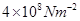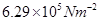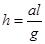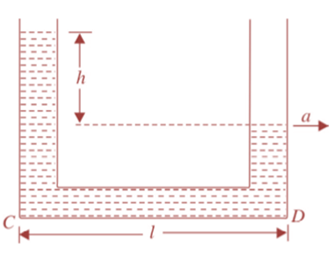# Mechanical Properties of Fluids(Pressure) test Paper

In this page we have Mechanical Properties of Fluids(Pressure) test Paper . Hope you like them and do not forget to like , social share and comment at the end of the page.

# Definition and derivation based questions

Question 1 Define term thrustand give its SI unit.
Question 2 Describe three practical applications from daily life which make use of concept of pressure.
Question 3 What do you mean by specific gravity or relative density of a substance.
Question 4 State and prove pascal’s law of transmission of fluid pressure.
Question 5 With the help of detailed diagram explain how Pascal’s law is applied in a hydraulic lift.
Question 6 Discuss the variation of fluid pressure with depth. Also explain how is Pascal’s law affected in the presence of gravity.
Question 7 Describe mercury barometer for measuring atmospheric pressure.
Question 8 Deduce an expression for apparent weight of a body immersed in a fluid.
Question 9 State and explain the law of flotation.  (give detailed answer)
Question 10 State the conditions for the equilibrium of floating bodies. Also discuss the stability of a floating body.

# Numerical based problems

Question 1 A force of 40 N is applied on a nail, whose tip has an area of cross-section of 0.001 cm2. Find the pressure on the tip.               (Answer:-)
Question 2 Torricelli’s barometer used mercury. Pascal duplicated it using French wine of density 984 Kgm-3. Determine the height of the wine column for normal atmospheric pressure.                      (Answer:-10.5 m)
Question 3 A hydraulic automobile lift is designed to lift cars with a maximum mass 3000 Kg. The area of cross-section of the piston carrying the load is 425 cm2. What maximum pressure would the smaller piston have to bear? (Answer:-)
Question 4 A sample of milk diluted with water has density of 1032 Kg-m-3. If pure milk has density 1080 Kg-m-3 , find the percentage of water by volume in milk.             (Answer:-60%)
Question 5 A pieces of ice floats in liquid in a beaker. What happens to the level of liquid in beaker, when ice melts completely?
Question 6 The density of ice is 917 Kg-m-3. What fraction of the volume of a piece of ice will be above water, when floating in fresh water? Density of water is 1000 Kg-m-3.  (Answer: - 0.083)
Question 7 The neck and bottom of a bottle are 2 cm and 10 cm in diameter respectively. If the cork is pressed with a force of 1.2 Kgf in the neck of the bottle, calculate the force exerted the bottom of the bottle.  (Answer:- 30 Kgf)

Question 8 A piece of metal of mass 17 g is tied to a cork of mass 5 g and the two remain suspended under water without sinking, when lowered into water. If the density of cork is 0.25 g-cm-3, find the density of metal. (Answer:- 8.5 g-cm-3)
Question 9 A body floats with 2/5 of its volume above the surface of water. Calculate the density of material of the body. (Answer:- 600 Kg-m-3)
Question 10 A liquid stands at the same level in the U-tube when at rest. If A is the area of cross-section of the tube and g is the acceleration due to gravity, what will be the difference in height of the liquid in the two limbs when the system is given acceleration ‘a’ towards the right as shown in Figure. (Answer:-)